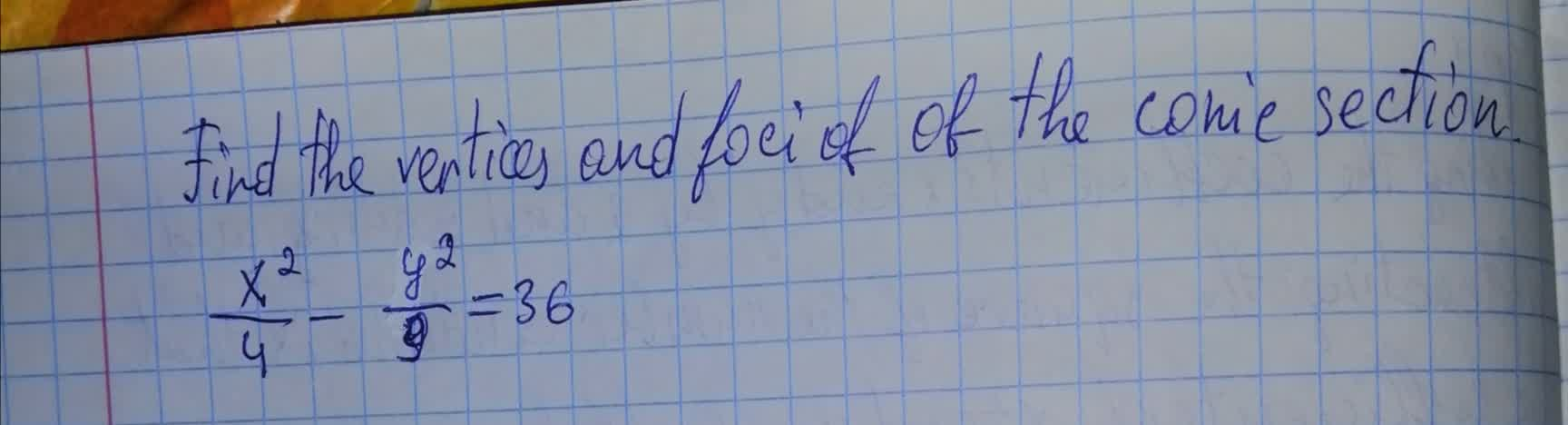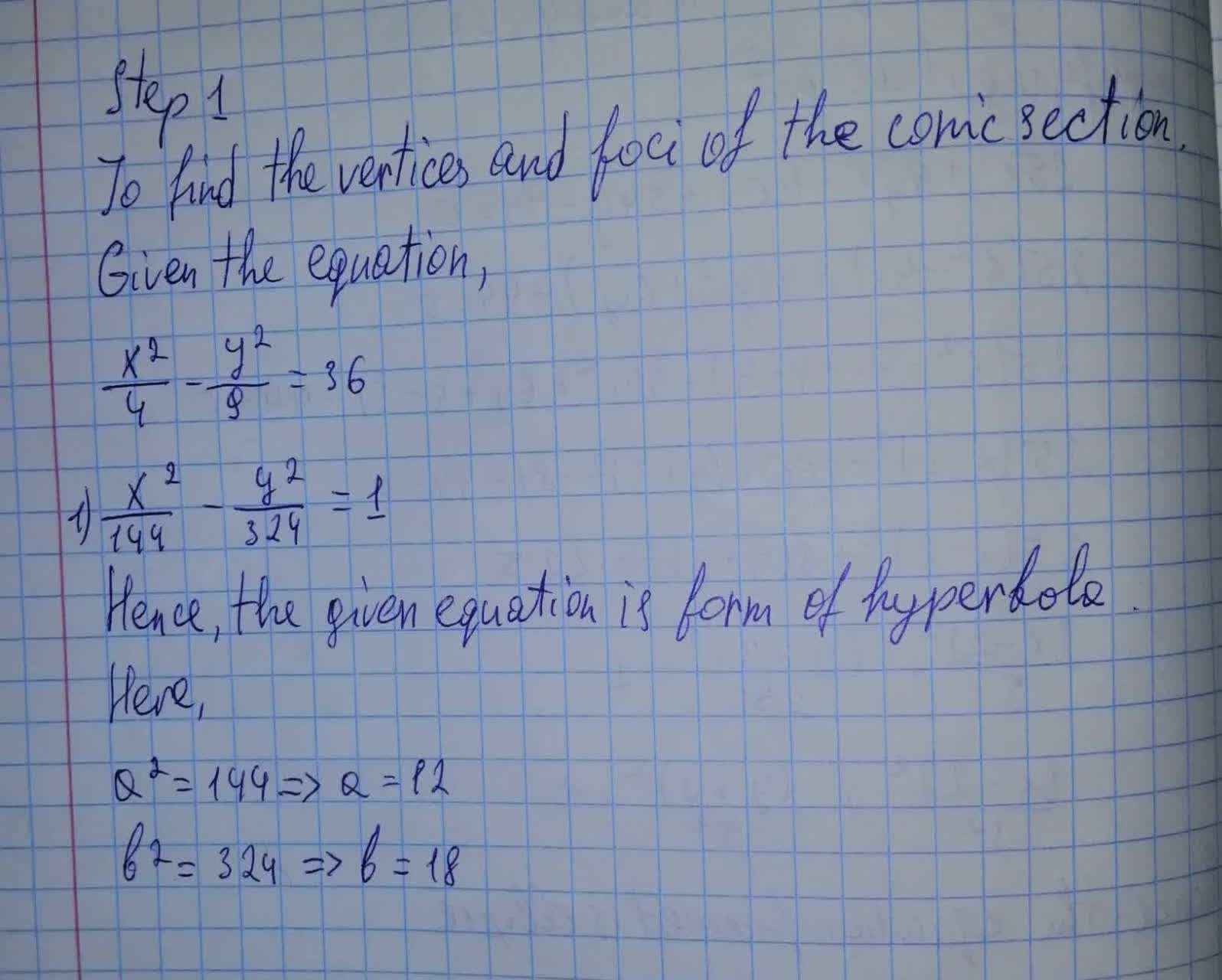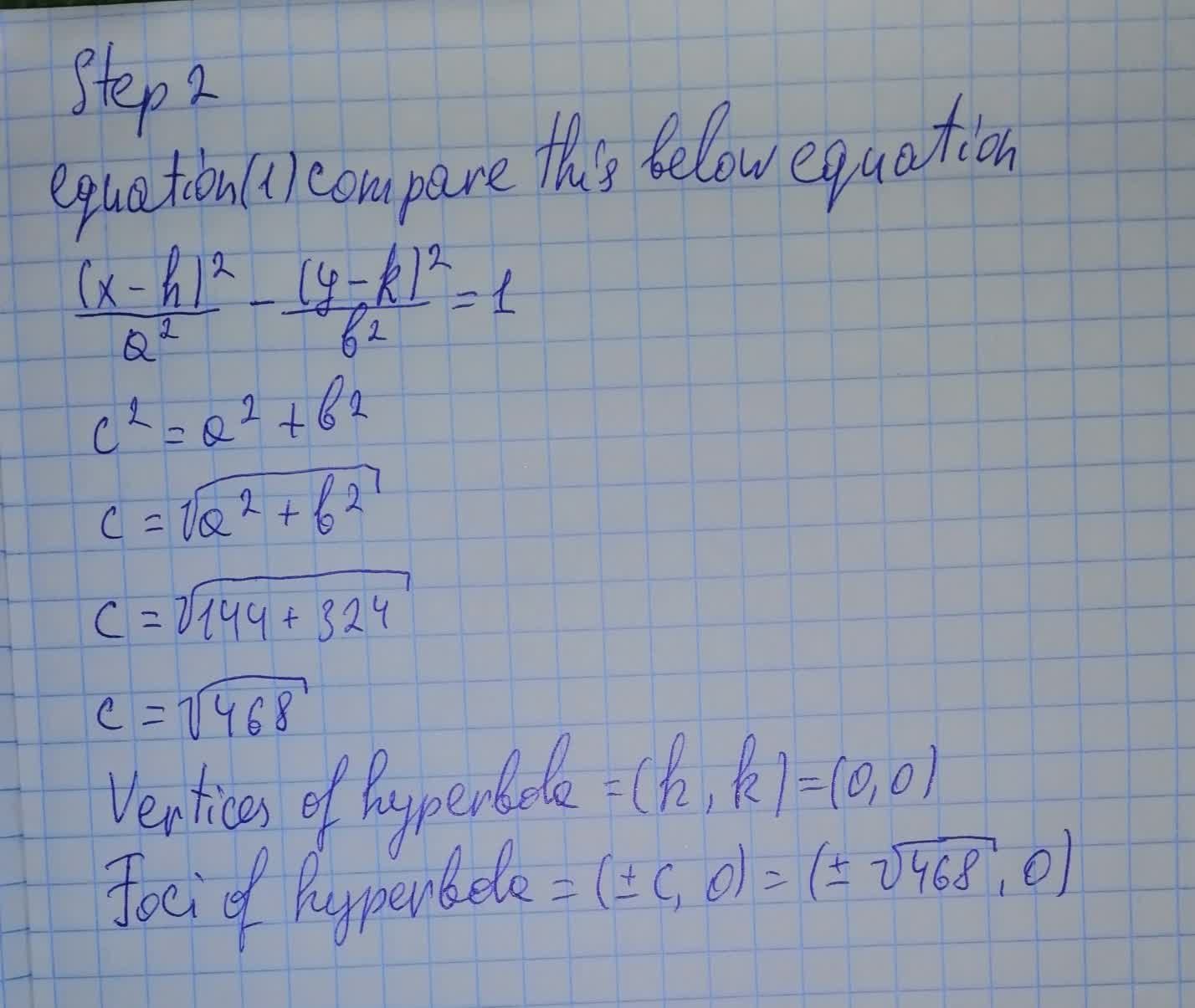# Find the vertices and foci of the conic section. x^2/4 − y^2/9 = 36tabita57i 2021-08-11 Answered

Find the vertices and foci of the conic section. $$\frac{x^2}{4 }− \frac{y^2}{9} = 36$$• Questions are typically answered in as fast as 30 minutes

### Plainmath recommends

• Get a detailed answer even on the hardest topics.
• Ask an expert for a step-by-step guidance to learn to do it yourself.Maciej Morrow

The conic section has solution: